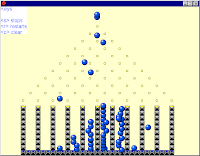### Collaborative discovery learning with the computerTitle: Collaborative mathematical investigations with the computer; learning materials and teacher help.
Author(s): M.H.J. Pijls
Publication type: PhD thesis

The PhD thesis that I have in front of me is on collaborative discovery learning using the computer.

Of course it is interesting to know whether education with computers gives better results than education without them. But that's not what this research is about: in all conditions the computer is used. In the first experiment described in this thesis it is investigated at which moment the computer can best be used: only in the beginning of the lesson cycle, during the whole lesson cycle or only at the end of the lesson cycle. The answer: for the test scores is doesn't matter. That one could also not use a computer at all doesn't seem to be a thought that crossed the researchers mind. In the second described experiment the computer was used during the whole lesson cycle.

Of course it's also interesting to investigate to what extent collaborative learning is effective. But this is also not done in this thesis. The researcher wonders whether it would have been better to divide the class into triples instead of into pairs, but that one could also divide the class into singles doesn't seem to have crossed her mind.

In the second experiment the effectiveness of 'process-help' versus 'product-help' is investigated. At first glance you might think that this means discovery versus instruction, but the following quote clearly indicates that it's not:

The learning materials contained no 'theory blocks' (i.e. sections in which a mathematical concept was shown and explained) and no 'correction sheets' with the correct answers to assess their work afterwards (the students were used to correct their work with the help of correction sheets after finishing the tasks). No classroom discussions took place in either project.

So both conditions are discovery learning. The only difference is that in the product-help condition the teacher was allowed to give the students (as pairs) mathematical hints and the process-help teacher was not allowed to talk about mathematics at all. Of course the product-help teacher encountered the problem with small-group learning: there is simply not enough time to give all the small groups the attention that they need. The teacher and the students therefore wanted to have 'whole class moments', but this was not allowed. On the post-test there was no difference between the process-help group and the product-help group. The researcher gave this the following spin (original in Dutch):

More explanation not always better.

Now let's take a closer look at the results. Maybe you know the game on the picture above. The students played games like this on the computer during the experiments. On one of the tests (it isn't mentioned whether it was the pre-test or the post-test) there was a question about this particular game (but with only 5 boxes at the bottom instead of the 10 that are shown in the picture above). The question was 'what is the probability that the ball will fall into the middle box?' There is a simple trick that helps to answer this (Pascal's triangle) and this was exactly the kind of question that was practiced during the 10 lesson experiment. There were 13 questions like this on the post-test for in total 46 points. The maximum number of points for this particular question was 4. For the (absolutely wrong) answer '1/5' a student got 1 point. For the correct but inaccurate answer 'more than 1/5' the student got 2 points. The average score of the students on the post-test was 14.29 points, that's an average of slightly more than 1 point per question. Since one apparently gets 1 point for a completely wrong answer to a question this is a very saddening score. In 10 lessons the students seem to have learned almost nothing. The researcher remarks the following about the improvement from pre-test to post-test:

The open-ended questions in this post-test were very much comparable to the pretest. The number of questions and the division of points were the same and the questions often had very similar contexts. This time, however, we expected the students to be able to make the majority of the tasks. [...] The difference between pre- and post-test in both conditions showed that on average all students' learning results improved (t-test for difference between pre- and post-test: t = 6.367, df = 51, p <.001).

This is 'on average' indeed true. From the information provided we can deduce that the average increase in score was 4.98 points with a standard deviation of 5.64 points. We can do some 'ballpark statistics' with this limited information provided. Assuming that the increase in scores is normally distributed we see that about 16% of the students has a negative increase. So our 'ballpark statistics' tells us that 9 of the 52 students knew less about this topic after the ten lessons on this topic then they knew before! However, this of course doesn't lead the researcher to question her computer-assisted collaborative discovery learning method...